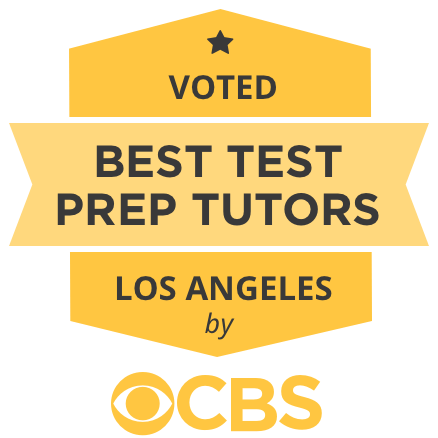1 of 7

# Award-Winning Private Statistics Tutoring in Los Angeles

CBS ranked us Best Tutors in LA! Contact us to hear why. We serve all of Southern Cal.

150,000+ clients. 4.9 / 5.0 Rating of Tutoring Sessions

### Private In-Home and Online Statistics Tutoring in Los Angeles, CA

Receive personally tailored Statistics lessons from exceptional tutors in a one-on-one setting. We help you connect with in-home and online tutoring that offers flexible scheduling and your choice of locations.Featured by
Tutors from

## 100% Tutor Satisfaction Guarantee

At Varsity Tutors, we're committed to connecting you to top tutors capable of delivering an outstanding experience. That's why we provide a 100% tutor satisfaction guarantee.

## How can a tutor assist you in conquering Statistics?

Statistics is an area of mathematics that focuses on learning from data. While many of the formulas used in statistics come from algebra, statistics gives the equations new meanings. With proper use of statistics, you can do an analysis of a set of data that predicts future trends. Before you get to this stage, however, you'll have to know the ins and outs of the topic. This may be more difficult than it sounds, but with the help of a statistics tutor in Los Angeles, California, found with the assistance of the educational directors at Varsity Tutors, you can work through your statistics course work.

Some of the most basic concepts in statistics are mean, average, and outliers. These equations come from algebra, but statistics gives the numbers behind them deeper meaning. But there is so much more you can learn about a set of data than just the average. With statistics, you can experiment on populations of data while accounting for predictable variables. This can give you your own data set to learn from. However, in order to have fun in statistics with your own experiments, you have to understand how to plan and conduct them properly. A tutor can work through a plan with you and discuss the pros and cons of each decision. Through statistics tutoring in Los Angeles, you can hone your experimentation plans and understanding of how statistics works.

## Session Summaries by Statistics TutorsDiscussed standard deviation, z-scores, percentiles, IQR, and boxplots. Completed worksheets regarding z-scores and constructing of data sets. We worked on a free response question for the AP Stats class. She was well prepared and asked great questions!Today, the student and I worked on chapter 14, which focused on correlations; which included finding the mean of x, the mean of y, the summation of x minus the mean of x, the summation of y minus the mean of y, the summation of x minus the mean of x squared, the summation of y minus the mean of y squared, the r, the a, and the b. We also worked on graphing correlations and determining the correlation coefficient from the graph. From the graph, we were also able to determine the regression equation. Further, we worked on analyzing a bivariate correlate on SPSS and then analyzing the output.Today the student and I met to discuss her Statistics assignment. She wanted to go over her homework involving the normal distribution. We worked through each of the problems, solving them using what she remembered from the lectures, the text book and the characteristics of the normal distribution. By the end of the assignment, I felt that the student had gotten a hand of solving the related items. The assignment was very well designed such that she was able to integrate previous problems to solve the ones later in the assignment. I basically explained the methods and then the student solved the problems. We plan to meet up weekly so that I can help her work through her assignments.